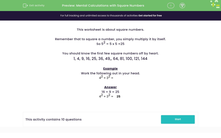# Do Calculations with Square Numbers Mentally

In this worksheet, students will add together square numbers in their heads.Key stage:  KS 3

Curriculum topic:   Number

Curriculum subtopic:   Understand Integer Powers/Real Roots

Popular topics:   Square Numbers worksheets

Difficulty level:#### Worksheet Overview

This activity is about square numbers.

Remember that to square a number, you simply multiply it by itself.

So 52 = 5 x 5 = 25

You should know the first few square numbers off by heart.

1, 4, 9, 16, 25, 36, 49,, 64, 81, 100, 121, 144

Example

42 + 32

16 + 9 = 25

42 + 32 = 25

Let's have a go at some questions now.

### What is EdPlace?

We're your National Curriculum aligned online education content provider helping each child succeed in English, maths and science from year 1 to GCSE. With an EdPlace account you’ll be able to track and measure progress, helping each child achieve their best. We build confidence and attainment by personalising each child’s learning at a level that suits them.

Get started